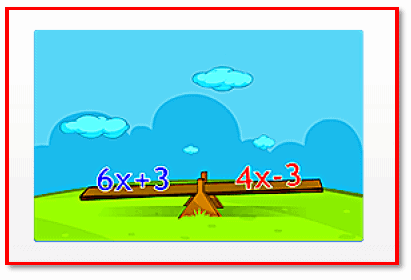Courses

# Chapter Notes - Simple Equations Class 7 Notes | EduRev

## Mathematics (Maths) Class 7

Created by: Praveen Kumar

## Class 7 : Chapter Notes - Simple Equations Class 7 Notes | EduRev

The document Chapter Notes - Simple Equations Class 7 Notes | EduRev is a part of the Class 7 Course Mathematics (Maths) Class 7.
All you need of Class 7 at this link: Class 7

Introduction to Simple EquationsAn equation is a condition of equality between two mathematical expressions.
Eg: 2x - 3 = 5
3x + 9 = 11
4y + 2 = 12
If the left hand side of an equation is equal to its right hand side for any value of the variable, then that value is called the solution of that equation.
Eg: For the equation, 5x + 5 = 15, x = 2 is a solution.
When we add or subtract the same number to or from both the sides of an equation, the value of the left hand side remains equal to its value on the right hand side.
Eg: 5x + 3 = 13
On subtracting 2 from both sides, we get 5x + 3 - 2 = 13 - 2 ; 5x + 1 = 11
When we divide or multiply an equation on both the sides by a non-zero number, the value of the left hand side remains equal to its value on the right hand side.
Eg: 5x + 1 = 13
- On dividing both sides by 4, we get (5x + 1)/4 = 13/4; 5x + 1 = 13
- On multiplying both sides by 4, we get 4(5x + 1) = 4(13); 20x + 4 = 52; 5x + 1 = 13

Application of Simple EquationsTo find the solution of an equation, we have to perform identical mathematical operations on the two sides of the equation so that only the variable remains on one side.
Eg: 3x + 8 = 84
3x + 8 - 8 = 84 - 8
3x = 76
Transposing means moving a term of the equation to the other side. Transposing a number is the same as adding or subtracting the same number from both sides of the equation.
Eg: To solve 2x + 8 = 24
Given, 2x + 8 = 24 Transposing 8 to the right hand side, we get ⇒ 2x = 24 - 8 ⇒ 2x = 16 x = 8
Hence, the value of x is 8.
When a number is transposed from one side of the equation to the other, its sign chang

Offer running on EduRev: Apply code STAYHOME200 to get INR 200 off on our premium plan EduRev Infinity!

,

,

,

,

,

,

,

,

,

,

,

,

,

,

,

,

,

,

,

,

,

;